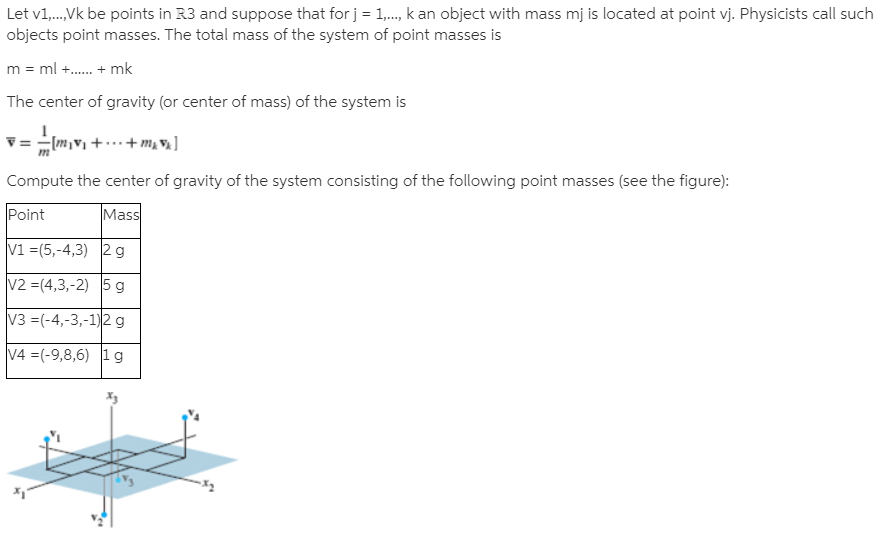# Let v1,.,Vk be points in R3 and suppose that for j = 1,., k an object with mass mj is located at point vj. Physicists call suchobjects point masses. The total mass of the system of point masses ism = ml +. + mkThe center of gravity (or center of mass) of the system isV = - (m,v + .+m, V ]Compute the center of gravity of the system consisting of the following point masses (see the figure):PointMassV1 =(5,-4,3) 2gV2 =(4,3,-2) 5 gV3 =(-4,-3,-1)2 gV4 =(-9,8,6) 1g

Question
1 viewshelp_outlineImage TranscriptioncloseLet v1,.,Vk be points in R3 and suppose that for j = 1,., k an object with mass mj is located at point vj. Physicists call such objects point masses. The total mass of the system of point masses is m = ml +. + mk The center of gravity (or center of mass) of the system is V = - (m,v + .+m, V ] Compute the center of gravity of the system consisting of the following point masses (see the figure): Point Mass V1 =(5,-4,3) 2g V2 =(4,3,-2) 5 g V3 =(-4,-3,-1)2 g V4 =(-9,8,6) 1g fullscreen

### This question hasn't been answered yet.